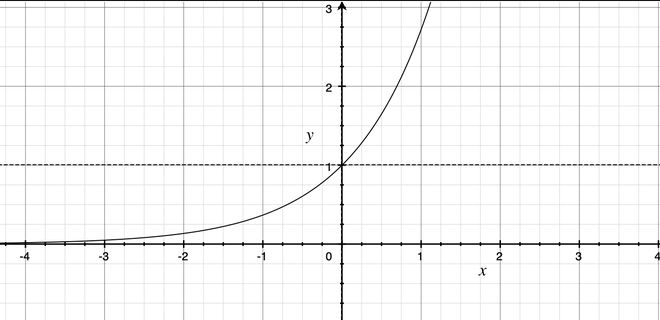Calculate exp(x) – 1 for all elements in a given NumPy array

• Difficulty Level : Medium
• Last Updated : 27 Aug, 2021

Exponential Function (e^x) is a mathematical function that calculates e raised to the power x where e is an irrational number, approximately 2.71828183.It can be calculated using the numpy.exp() method. This mathematical function helps user to calculate exponential of all the elements in the input array.

Syntax: numpy.exp(arr, out, where)

Parameters:
arr : Input
out : A location into which the result is stored. If provided, it must have a shape that the
inputs broadcast to. If not provided or None, a freshly-allocated array is returned.
shape must be same as input array.
where : Boolean Value.True value means to calculate the universal functions(ufunc) at that position, False value means to leave the value in the output alone.

If a scalar is provided to the function as input then the function is applied on the scalar and another scalar is returned.

Example 1: If 3 was given as input then e^3 will returned as output.

Python

 import numpy n = 4print(numpy.exp(n)) n = 5print(numpy.exp(n))

Output :

54.598150033144236
148.4131591025766

If input is an array then function is applied element-wise. ex- np.exp([1,2,3]) is equivalent to [np.exp(1),np.exp(2),np.exp(3)]

Method 1: Iterating over array

Python

 # importing numpyimport numpy  arr = [1, 2, 3, 4] print("Input : ", arr)for i in range(len(arr)):    arr[i] = numpy.exp(arr[i])-1 print("Output : ", arr) arr = [3, 0.3, 3.1, 2.2] print("Input : ", arr)for i in range(len(arr)):    arr[i] = numpy.exp(arr[i])-1 print("Output : ", arr)

Output:

Input :  [1, 2, 3, 4]
Output :  [1.718281828459045, 6.38905609893065, 19.085536923187668, 53.598150033144236]
Input :  [3, 0.3, 3.1, 2.2]
Output :  [19.085536923187668, 0.3498588075760032, 21.197951281441636, 8.025013499434122]

Method 2: Providing array as input to numpy.exp() function

Python

 # importing numpyimport numpy arr = [1, 2, 3, 4] print("Input : ", arr) arr = numpy.exp(arr)-1print("Output : ", arr)  arr = [3, 0.3, 3.1, 2.2] print("Input : ", arr) arr = numpy.exp(arr)-1print("Output : ", arr)

Output:

Input :  [1, 2, 3, 4]
Output :  [ 1.71828183  6.3890561  19.08553692 53.59815003]
Input :  [3, 0.3, 3.1, 2.2]
Output :  [19.08553692  0.34985881 21.19795128  8.0250135 ]

My Personal Notes arrow_drop_up